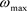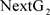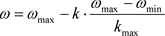﻿ 一种混合粒子群算法求解Nash平衡

# 一种混合粒子群算法求解Nash平衡A Hybrid Particle Swarm Optimization for Solving Nash Equilibrium

Abstract: At present, many intelligent algorithms have been used for solving the Nash equilibrium. They have their own advantages and disadvantages. To overcome local optimum, it designs a hybrid Particle Swarm Optimization for solving Nash equilibrium, by combining the crossover operator in Genetic Algorithm with the basic Particle Swarm Optimization. Experiments show that the algorithm designed is effective, and it is superior to the immune algorithm, immune Particle Swarm Optimization, and basic Particle Swarm Optimization.

1. 引言

Nash证明了n人非合作博弈Nash平衡的存在性 ，但迄今未有通用算法来求解所有的博弈模型。Nash平衡的求解，最终可归结为求解一个约束优化问题，要得到此优化问题的准确解通常是很难的。然而在许多实际问题中，我们必须得到此优化问题的一个具体的解。一般采用某些数值方法求其近似，但数值方法通常要求可导、连续等条件。另一类方法是采用智能算法，这些算法直接对结构对象进行操作，不要求可导、连续等条件，它们具有内在的并行性和较好的寻优能力，可以自动调整搜索方向，从而较好地得到优化问题的解。目前，已有多种智能算法用来求解Nash平衡，包括遗传算法 、启发式搜索算法 、免疫算法 、粒子群算法  及一些改进的粒子群算法   等。

2. 问题描述

$f\left(x,y\right)=\mathrm{max}\left\{\underset{1\le i\le m}{\mathrm{max}}\left\{{A}_{i}{y}^{\text{T}}-xA{y}^{\text{T}}\right\},0\right\}+\mathrm{max}\left\{\underset{1\le i\le n}{\mathrm{max}}\left\{x{B}_{j}-xB{y}^{\text{T}}\right\},0\right\}$

3. 混合粒子群算法设计

${v}_{i}^{k+1}=\omega {v}_{i}^{k}+{c}_{1}{r}_{1}\left({p}_{i}-{x}_{i}^{k}\right)+{c}_{2}{r}_{2}\left({p}_{g}-{x}_{i}^{k}\right)$ (1)

${x}_{i}^{k+1}={x}_{i}^{k}+{v}_{i}^{k+1}$ (2)

Step 1确定学习因子 ${c}_{1},{c}_{2}$，群体规模N，最大迭代代数 ${k}_{\mathrm{max}}$${\omega }_{\mathrm{min}}$ 及精确度 $ϵ$

Step 2随机初始化粒子群及其速度，使每个粒子的第i个向量满足

${x}_{1}^{i}+{x}_{2}^{i}+\cdots +{x}_{{m}_{i}}^{i}=1,\text{\hspace{0.17em}}{x}_{j}^{i}\ge 0,\text{\hspace{0.17em}}j=1,2,\cdots ,{m}_{i},i=1,2,\cdots ,N$

Step 3计算各个粒子的适应度，并对粒子按照适应度大小进行排序，适应度小的一半粒子直接进入下一代 ${\text{NextG}}_{1}$ (另一半记为 ${\text{NextG}}_{2}$ )，对适应度大的一半粒子进行杂交，杂交之后先对粒子作归一化处理，使之满足 ${x}_{1}^{i}+{x}_{2}^{i}+\cdots +{x}_{{m}_{i}}^{i}=1,{x}_{j}^{i}\ge 0,j=1,2,\cdots ,{m}_{i},i=1,2,\cdots ,N$ ；并与形成粒子池，计算粒子池中粒子的适应度，选择适应度小的一半粒子与 ${\text{NextG}}_{1}$ 形成下一代粒子 $\text{NextG}$

Step 4计算当前粒子群 ${P}_{k}$ 中粒子的适应度值及 ${p}_{i}\left(i=1,\cdots ,N\right)$， 并把 ${p}_{g}$ 作为记忆粒子存入记忆库中。

Step 5判断是否满足 $f\left({p}_{g}\right)<ϵ$$k\ge {k}_{\mathrm{max}}$。 若满足，则停止并输出迭代次数 $k,\text{\hspace{0.17em}}{p}_{g}$ 以及 ${p}_{g}$ 的适应值;否则，继续。

Step 6利用公式计算惯性权重 $\omega$

Step 7按公式(1)与(2)更新 ${P}_{k}$ 中粒子的速度与位置。

Step 8依次检验第i个粒子 ${x}_{i}^{k+1}$ 是否属于 ${x}_{i}^{k+1}>0$。否则，计算控制步长 ${\alpha }_{i}$ (此技术来自文献 )，并令 ${x}_{i}^{k+1}={x}_{i}^{k}+{\alpha }_{i}\cdot {v}_{i}^{k+1}$，然后作归一化处理，使得 ${x}_{i}^{k+1}$ 回到混合策略组合空间中，形成新一代粒子群体 ${P}_{k+1}$。然后转到第3步。

4. 数值例子Table 1. Computing result of game Γ ( A 1 , B 1 )Table 2. Computing result of game Γ ( A 2 , B 2 )Table 3. Computing result of game Γ ( A 3 , B 3 )Table 4. Computing result of game Γ ( A 4 , B 4 )Table 5. Computing result of game Γ ( A 5 , B 5 )Table 6. Computing result of game Γ ( A 6 , B 6 )

5. 结束语

 卿东升, 邓巧玲, 李建军, 刘帅, 刘鑫, 曾素平. 基于粒子群算法的满载需求可拆分车辆路径规划[J]. 控制与决策, 2020, 35(2): 1-9.

 梁静, 葛士磊, 瞿博阳, 于坤杰. 求解电力系统经济调度问题的改进粒子群优化算法[J]. 控制与决策, 2019, 34(3): 1-10.

 唐红亮, 吴柏林, 胡旺, 康承旭. 基于粒子群优化的地震应急物资多目标调度算法[J]. 电子与信息学报, 2020, 42(3): 737-745.

 李倩. 粒子群优化算法在港口船舶物流中的应用[J]. 舰船科学技术, 2020, 42(2): 205-207.

 余谦, 王先甲. 基于粒子群优化求解纳什均衡的演化算法[J]. 武汉大学学报, 2006, 52(1): 25-29.

 Nash, J. (1951) Non-Cooperative Games. The Annals of Mathematics, 54, 286-295.
https://doi.org/10.2307/1969529

 陈士俊, 孙永广, 吴宗鑫. 一种求解Nash均衡解的遗传算法[J]. 系统工程, 2001(19): 67-70.

 隗立涛, 修乃华. 基于启发搜索算法的纳什均衡计算[J]. 北京交通大学学报, 2001, 40(33): 87-92.

 邱中华, 高洁, 朱跃星. 应用免疫算法求解博弈问题[J]. 系统工程学报, 2006, 21(4): 398-404.

 刘露萍, 贾文生, 蔡江华. 协同免疫量子粒子群算法求非合作博弈Nash平衡[J]. 计算机应用于软件, 2019(8): 203-209.

 贾文生, 向淑文, 杨剑锋, 等. 基于免疫粒子群算法的非合作博弈Nash均衡问题求解[J]. 计算机应用研究, 2012, 29(1): 28-31.

 汪贤裕, 肖玉明. 博弈论及其应用[M]. 北京: 科学出版社, 2008: 15-48.

 汪定伟, 王俊伟, 王洪峰, 等. 智能优化方法[M]. 北京: 高等教育出版社, 2007: 217-252.

Top